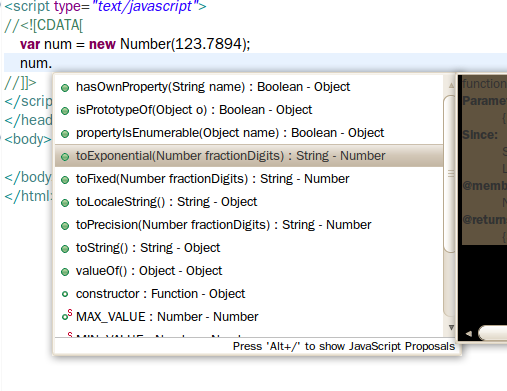# Number

If you work in Eclipse, you can get the code assist as shown below.```var num = new Number(2194.123456);
alert("A. "+num.toExponential(3)); //Exponential representation of the part beyond three decimal places
alert("B. "+num.toPrecision(3)); //3 significant digits
alert("C. "+num.toFixed(8)); //8 decimal places
alert("D. "+num.toLocaleString()); //Locale digits
alert("E. "+num.toString()); //Return as a string
alert("F. "+Number.MAX_VALUE); //max value
alert("G. "+Number.MIN_VALUE); //minimum value
alert("H. "+Number.NEGATIVE_INFINITY);
alert("I. "+Number.POSITIVE_INFINITY);
alert("J. "+Number.NaN); //Not a Number
alert("K. "+(num.valueOf() + 1)); //Returns the value of the Number object as a primitive value
//The isNaN() intrinsic is related to the number and is introduced here.
if (isNaN("two thousand eighteen") == true) {
alert("L. Not a Number");
}
```

There is a 'prototype' property.
The 'prototype' property allows you to add a new property or method to the object.